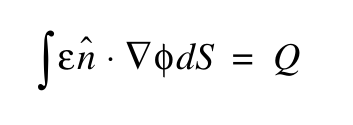# How to simulate a floating metal contact?

I would like to simulate a floating metal contact inside an insulator in my device.

The surface potential should be a constant that satisfy the Gauss’s law:where Q is total charge of the floating contact.

Let’s say I have already created the edge model “EdgeFlux”, equal to “Permittivity * (Potential@n0 - Potential@n1) * EdgeInverseLength”.
How shall I create the contact equation to enforce such boundary condition?
I looked into the contact_equation command but haven’t figured out a method.

Hi @KKwok

Thanks for your question. My idea would be to:

1. Create an external node
2. Attach a current source to this node with the magnitude of the charge

For the Electron and Hole contact equations, do not contribute to these options:

``````circuit_node=""
node_charge_model=""
edge_charge_model=""
node_current_model=""
edge_current_model=""
``````

For the Potential equation, set

``````circuit_node=GetContactBiasName(contact)
edge_current_model=contactedgecharge_edge
edge_charge_model=""
node_current_model=""
node_charge_model=""
``````

In essence, you are treating the charge as if it was a current. Please let me know if you have any further questions.

The `contactcharge_edge` model is defined in `python_packages/__init__.py`.

``````        contact_equation(device=device, contact=contact, name="ElectronContinuityEquation",
node_model=contact_electrons_name,
edge_current_model="", circuit_node="")

contact_equation(device=device, contact=contact, name="HoleContinuityEquation",
node_model=contact_holes_name,
edge_current_model="", circuit_node="")

contact_equation(device=device, contact=contact, name="PotentialEquation",
node_model=contact_model_name, edge_current_model= contactcharge_edge
circuit_node=GetContactBiasName(contact)
)

``````

Thank you. It works!

1 Like

I’m glad. I appreciate the update